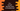# C++ program to convert a decimal value to hexadecimal## How to convert a decimal value to hexadecimal:

Decimal numbers are numbers with base value 10 and hexadecimal numbers are numbers with base 16. Since hexadecimal is a base 16 number format, 0 to 9 are represented by 0 to 9 and 10 to 15 are represented by A to F.

### How to convert decimal to hexadecimal:

To convert a decimal value to hexadecimal value, we need to keep dividing the decimal value by 16 until we reach 0. On each step, we will pick the remainder. Using the remainders, we can build the final hexadecimal conversion.

For example, Let’s try to convert 2000 to hexadecimal:

• Divide 2000 by 16, 2000/16 = 125, remainder - 0
• 125/16 = 7, remainder - 13
• 7/16 = 0, remadinder - 7

We have the remainders : 7, 13 and 0 from bottom to top. The hexadecimal value of 13 is D. So, the hexadecimal value of 2000 is 7D0.

### Algorithm for a C++ program to convert a decimal value to hexadecimal:

Since we have both numbers and characters to store for a hexadecimal value, we need to store them in a character array. For that, we need to convert the integer value to character and it can be done by storing the ASCII values. ASCII value of 0 is 48 and ASCII value of A is 65.

So, if the current remainder is less than 10, we can add it to 48 and store that in the character array. And, if it is more than 10, we can add it to 65 to convert the value to the character that we want and store that value in the character array.

For example, if it is 12, we will add 55 and it will become 67, which is ASCII of C.

### C++ program to convert a decimal value to hexadecimal:

Below is the complete C++ program to convert decimal to hexadecimal:

``````#include <iostream>
#include <algorithm>

using namespace std;

string decimalToHexa(int no)
{
char hexa;
int position = 0;
while (no != 0)
{
int currentValue = no % 16;

if (currentValue < 10)
{
hexa[position] = currentValue + 48;
position++;
}
else
{
hexa[position] = currentValue + 55;
position++;
}

no = no / 16;
}

string hexa_string = string(hexa);
reverse(hexa_string.begin(), hexa_string.end());

return hexa_string;
}

int main()
{
cout << decimalToHexa(2000) << endl;
}``````

Here,

• decimalToHexa method is used to convert a decimal value to hexadecimal. This method takes one integer value as argument and returns one string i.e. the hexadecimal value as string.
• Inside decimalToHexa, we are using a while loop to keep dividing the number by 16. The result is put in a different array called hexa.
• Once the while loop is completed, the result is converted to an array using string()
• The result, i.e. the values are in reverse order. So, we used reverse() function to reverse the result string.
• This string is returned back.

If you run the above program, it will print the below output:

``7D0``